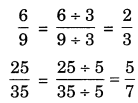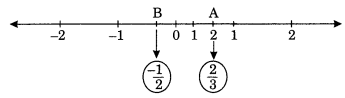On this page, you will find Rational Numbers Class 7 Notes Maths Chapter 9 Pdf free download. CBSE NCERT Class 7 Maths Notes Chapter 9 Rational Numbers will seemingly help them to revise the important concepts in less time.

## CBSE Class 7 Maths Chapter 9 Notes Rational Numbers

### Rational Numbers Class 7 Notes Conceptual Facts

1. Rational numbers: The number which are in the form of $$\frac{p}{q}$$ where p and q are co-prime and
q ≠ 0 are called rational numbers.

2. All integers and fractions are rational numbers.

3. When we compare two integers, we need rational numbers.
e.g. 2 : 3 = $$\frac{2}{3}$$ a rational number.

4. 0 is a rational number.

5. A rational number is said to positive if both of the numerator and denominator are either positive or negative.
$$\text { e.g. } \frac{5}{6}, \frac{-2}{-3}, \frac{0}{2} \text { etc }$$

6. A rational number is said to be negative if one of the numerator or denominator is negative.
$$\text { e.g. } \frac{-1}{2}, \frac{3}{-5}, \frac{0}{-1} \text { etc. }$$

7. Every integer is a rational number but every rational number need not to be an integer.

Properties of rational numbers:
(i) Equivalence of rational numbers: If $$\frac{p}{q}$$ is a rational number and m is a not zero integer, then(ii) Reducting a rational number to its simplest form: a rational number and m is a common p+m r divisor top and q then $$\frac{p}{q}$$, where H.C.F. of r and s is 1.Standard form of a rational number: A rational number is said to be in standard form if its denominatorRational numbers between two rational numbers:
There are unlimited rational numbers between two rational numbers.

Rational numbers on a number line.

• Mark a point O on a straight line already marked with arrows at its end points.
• Mark points on the line at unit length interval from each other on both sides like 1, 2, 3, … on right side of 0 and -3,-2, 1 on its left side.
• To represent rational number $$\frac{2}{3} \text { and }-\frac{1}{2}$$ on a number line.Since $$\frac{2}{3}$$ < 1
∴ Divide the first unit into three equal parts and mark division 2 by A which represent a rational 2
number $$\frac{2}{3}$$. Similarly, divide the first unit on the left into two equal parts. Mark the middle one
3 1 by B which represents a rational number –$$\frac{2}{3}$$.

Absolute value of a rational number: The absolute value of a rational number |a| is written as which shows its numerical value only regardless of its sign.
eg,…